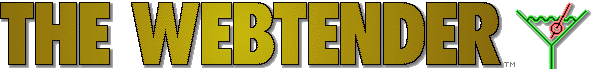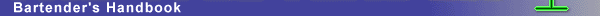# Bar Measurements

### Standard Bar Measurements (US)

 1 part = any equal part 1 dash/splash = 1/32 ounce 1 teaspoon (tsp) = 1/8 ounce 1 tablespoon (tblsp) = 3/8 ounce 1 pony = 1 ounce 1 jigger/bar glass = 1 1/2 ounces 1 shot (*) = 1 1/2 ounces 1 snit = 3 ounces 1 wineglass = 4 ounces 1 split = 6 ounces 1 cup = 8 ounces 1 pint (pt) = 16 ounces 1 quart (qt) = 32 ounces 1 fifth = 25.6 ounces (1/5 gallon) 1 gallon (gal) = 128 ounces

### Metric Conversions

 1 fluid ounce (oz) = 29.573 milliliters = appx. 3 centiliters (cl) 1 quart (qt) = 9.4635 deciliters 1 gallon (gal) = 3.7854 liters 1 milliliter (ml) = 1/30 ounce 1 centiliter (cl) = 1/3 ounce 1 deciliter (dl) = 3 1/2 ounces 1 liter (l) = 34 ounces

### Other Measurements

English Metric
Fifth = 4/5 Quart = 1/5 Gal. = 25.6 oz    =>    750 ml = 25.5 oz
Pint (pt) = 1/2 Quart = 16.0 oz    =>    500 ml = 17.0 oz
Half-Pint = 8.0 oz    =>    200 ml = 6.8 oz
Half-Gallon = 64.0 oz    =>    1750 ml = 59.7 oz
Quart = 32.0 oz    =>    1000 ml = 34.1 oz

(*) A "shotglass" is usually 1.5 ounces, but sometimes 2 ounces with a measuring line at 1.5 ounces. You can also buy (in US) "short shot" glasses or "pony shots" which are 1 ounce. Pony shots are usually used with martinis, manhattans, and rob roys.

 Do you know a good web page related to drinks, bartending, wine or similar subjects? Visit The Webtender Index and add it!

Home · Drink Recipes · Forums · Bookstore · Barstore · Web Index · Feedback